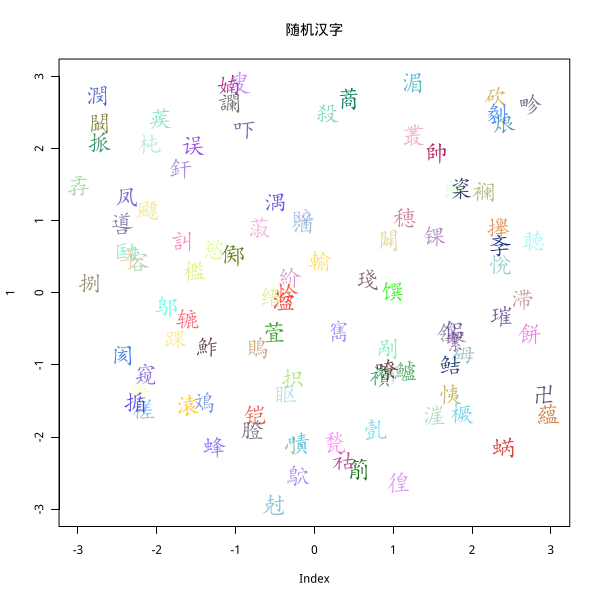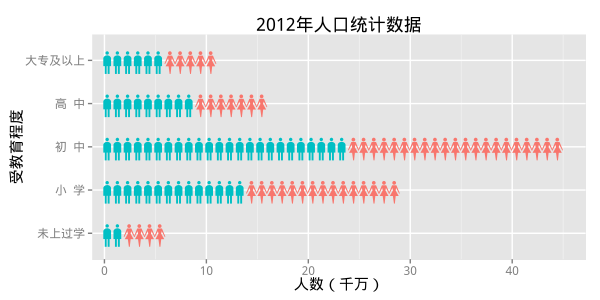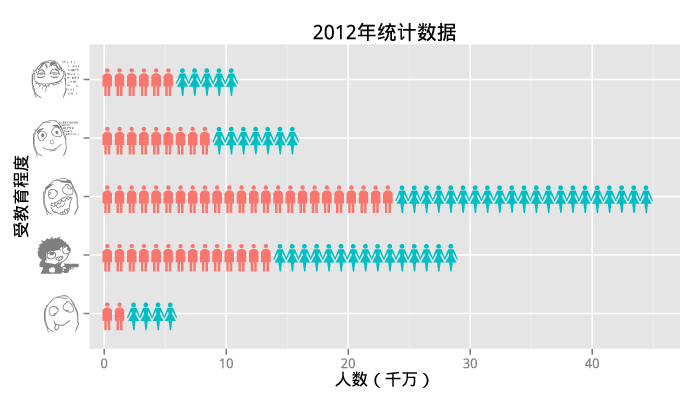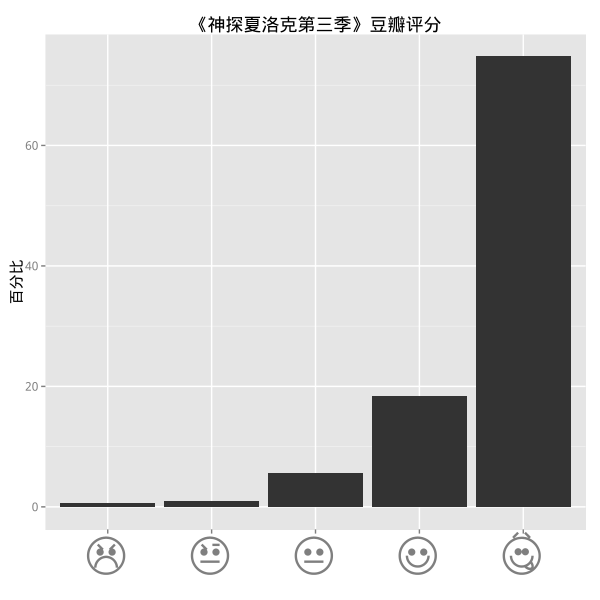# 使用字体

font.add(family, regular, ...)


font.add("kaishu", "simkai.ttf")


showtext的用法更加简单，目前只有两个函数：showtext.begin()showtext.end()。我们需要做的就是把绘图的命令包含在这两个语句中间，然后在适当的地方选取字体即可。不多说，直接上代码：

# showtext会自动加载sysfonts包
library(showtext);
# 导入楷体

library(Cairo);
# 打开图形设备
CairoPNG("chinese-char.png", 600, 600);
# 开始使用showtext
showtext.begin();
# 一系列绘图命令
set.seed(123);
plot(1, xlim = c(-3, 3), ylim = c(-3, 3), type = "n");
text(runif(100, -3, 3), runif(100, -3, 3),
intToUtf8(round(runif(100, 19968, 40869)), multiple = TRUE),
col = rgb(runif(100), runif(100), runif(100), 0.5 + runif(100)/2),
cex = 2, family = "kaishu");    # 指定kaishu字体
title("随机汉字", family = "wqy");   # 指定wqy字体
# 停止使用showtext
showtext.end();
# 关闭图形设备
dev.off();（我赌两块糖，你不认识上面一半以上的汉字……）

# 好玩的字体link = "http://img.dafont.com/dl/?f=wm_people_1";
unzip("wmpeople1.zip");

library(showtext);

library(ggplot2);
library(plyr);
library(Cairo);

edu,educode,gender,population

'));

dat$int = round(dat$population / 10000);
gdat = ddply(dat, "educode", function(d) {
male = d$int[d$gender == "m"];
female = d$int[d$gender == "f"];
data.frame(gender = c(rep("m", male), rep("f", female)),
x = 1:(male + female));
});
gdat$char = ifelse(gdat$gender == "m", "p", "u");

CairoPNG("edu-stat.png", 600, 300);
showtext.begin();
theme_set(theme_grey(base_size = 15));
ggplot(gdat, aes(x = x, y = educode)) +
geom_text(aes(label = char, colour = gender),
family = "wmpeople1", size = 8) +
scale_x_continuous("人数（千万）") +
scale_y_discrete("受教育程度",
labels = unique(dat$edu[order(dat$educode)])) +
scale_colour_hue(guide = FALSE) +
ggtitle("2012年人口统计数据");
showtext.end();
dev.off();


# 好玩的图形link = "http://img.dafont.com/dl/?f=emoticons";
unzip("emoticons.zip");

library(showtext);

library(ggplot2);
library(Cairo);
emotions = c("W", "s", "C", "A", "p");
score = c(0.5, 0.9, 5.5, 18.4, 74.7);
x = factor(emotions, emotions);
gdat2 = data.frame(x, score);
CairoPNG("douban.png", 600, 600);
showtext.begin();
ggplot(gdat2, aes(x = x, y = score)) +
geom_bar(stat = "identity") +
scale_x_discrete("") +
scale_y_continuous("百分比") +
theme(axis.text.x=element_text(size=rel(4), family="emoticons")) +
ggtitle("《神探夏洛克第三季》豆瓣评分");
showtext.end();
dev.off();